Win up to 100% scholarship on Aakash BYJU'S JEE/NEET courses with ABNAT Win up to 100% scholarship on Aakash BYJU'S JEE/NEET courses with ABNAT

# Electric Field

An Electric field can be considered an electric property associated with each point in the space where a charge is present in any form. An electric field is also described as the electric force per unit charge.

The formula of the electric field is given as,

E = F /Q

Where,

E is the electric field.
F is the force.
Q is the charge.

Electric fields are usually caused by varying magnetic fields or electric charges. Electric field strength is measured in the SI unit volt per metre (V/m).

The direction of the field is taken as the direction of the force which is exerted on the positive charge. The electric field is radially outwards from the positive charge and radially towards the negative point charge.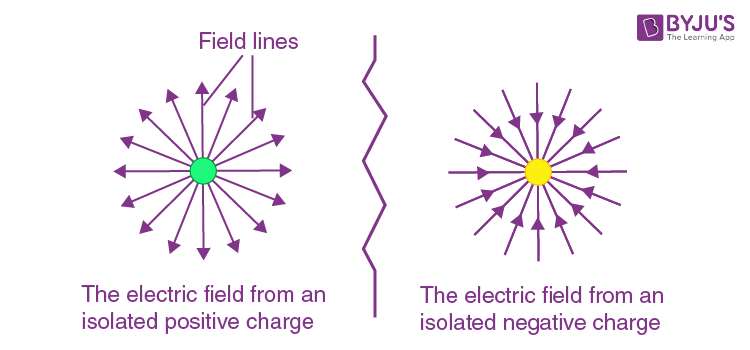## What Is an Electric Field?

An electric field is defined mathematically as a vector field that can be associated with each point in space, the force per unit charge exerted on a positive test charge at rest at that point.

The electric field is generated by the electric charge or by time-varying magnetic fields. In the case of an atomic scale, the electric field is responsible for the attractive forces between the atomic nucleus and electrons which hold them together.

According to Coulomb’s law, a particle with electric charge q1 at position x1 exerts a force on a particle with charge q0 at position x0 of,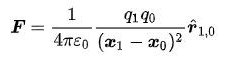Where,

r1,0 is the unit vector in the direction from point x1 to point x0

ε0 is the electric constant, also known as absolute permittivity of free space C2m-2N-1

When the charges q0 and q1 have the same sign, the force is positive, and the direction is away from other charges, which means they repel each other. When the charges have unlike signs,  the force is negative, and the particles attract each other.

The electric field is force per unit charge,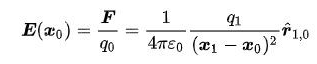The electric field can be found easily by using Gauss’s law which states that the total electric flux out of a closed surface is equal to the charge enclosed divided by the permittivity.

Or total flux linked with a surface is 1/ ε0 times the charge enclosed by the closed surface.

$$\begin{array}{l}\oint \vec{E}\cdot \vec{d}s = \frac{1}{\epsilon _{o}}q\cdot\end{array}$$

The electric field can also be calculated by Coulomb’s law, but Gauss’s law is easier. Besides, Gauss’s law is just a replica of Coulomb’s law. If we apply Gauss’s theorem to a point charge enclosed by a sphere, we will arrive at Coulomb’s law.

## How to Find Electric Field Using Gauss’s Law?

These include a few steps.

1. Initially, we should find the spatial symmetry (spherical, cylindrical, planar) of charge distribution.
2. Next, we need to find the Gaussian symmetry, same as that of the symmetry of spatial arrangement.
3. Find the integral along the Gaussian surface and then find the flux.
4. Find charge enclosed by Gaussian surface.
5. Find the electric field of charge distribution, the electric field due to a point charge.

The electric field is a vector field which is associated with the Coulomb force experienced by a test charge at each point in the space to the source charge. The magnitude and the direction of the electric field can be determined by the Coulomb force F on the test charge q. If the field is created by a positive charge, the electric field will be in a radially outward direction, and if the field is created by a negative charge, the electric field will be in a radially inwards direction.

Let a point charge Q be placed in a vacuum. Then, if we introduce another point charge q (test charge) at a distance r from the charge Q,Then, the electric field at point p due to the point charge Q is given by,

$$\begin{array}{l}\vec{E} = \frac{\vec{F}}{Q}\end{array}$$

The direction of the electric field due to a point charge Q is shown in the above figure. The magnitude of the electric field is proportional to the length of E. If a test charge, which is relatively larger, is brought within the area of the source charge Q, it is bound to modify the original electric field due to the source charge. A simple way to escape from this conflict is to use a very negligible test charge q.

Then, our definition of electric field modifies to,

$$\begin{array}{l}\vec{E} = \frac{lim}{q\rightarrow 0}(\frac{\vec{F}}{Q})\end{array}$$

According to this definition,

The electric field at point P due to point charge Q is,

$$\begin{array}{l}\vec{E} = \frac{1}{4\pi \epsilon o} \frac{Q}{r^{2}} \hat{r}\end{array}$$

## Electric Field Due to Line Charge

One application of Gauss’s law is to find the electric field due to the charged particle. Electric field due to line charge can be found easily by using Gauss’s law. Consider,

A line charge is in the form of a thin charged rod with linear charge density λ.To find the electric intensity at point P at a perpendicular distance r from the rod, let us consider a right circular closed cylinder of radius r and length l with an infinitely long line of charge as its axis.

The magnitude of the electric field intensity at every point on the curved surface of the Gaussian surface (cylinder) is the same since all points are at the same distance from the line charge.

Therefore, the contribution of the curved surface of the cylinder towards electric flux,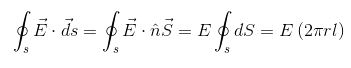2 Π rl is the curved surface area of the cylinder.

On the ends of the cylinder, the angle between the electric field and its direction is 90o. So, these ends of the cylinder will not have any effect on the electric flux.

Therefore,

ϕ E =q/ εo

Charge enclosed in cylinder=line charge density × length= λ l, so according to Gauss’s law,

ϕ E =q/ εo

E(2 Π rl)= λ l/ εo

Or,

E= λ /(2 Π εor)

## Electric Field Due to Ring

We want to find the electric field at an axial point P due to a uniformly charged ring, as shown in the figure: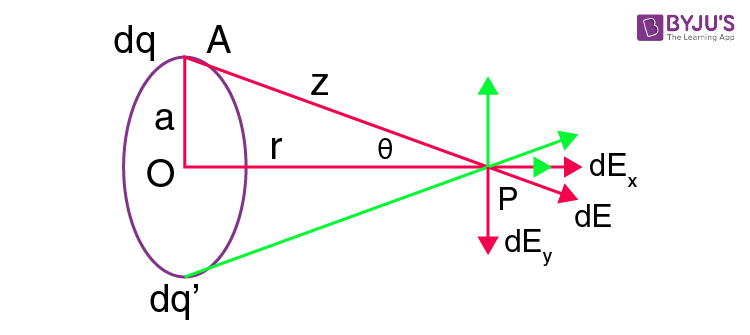The centre of the ring is at point O.

The circumference of the circle makes an angle θ with line OP drawn from the centre of the ring to point P.

Now, consider a small element from the ring as dq, and calculate the field at point P due to this charge element.

We know that the electric field at point P is,

$$\begin{array}{l}dE = \frac{dq}{4\pi \epsilon oz^{2}}\end{array}$$

The direction of the field is along AP. So, by resolving it, we get one along the positive x-direction and another along the negative y-direction, as shown in the above figure.

The x component of the electric field due to charge element dq is,

$$\begin{array}{l}dE_{x} = dEcos(\Theta) = \frac{dq}{4\pi \epsilon oz^{2}} cos (\Theta )\end{array}$$

The y component of the electric field due to charged element dq is,

$$\begin{array}{l}dE_{x} = dEsin(\Theta) = \frac{dq}{4\pi \epsilon oz^{2}} sin (\Theta )\end{array}$$

To calculate the total electric field at point P due to the charge ring, we need to integrate dE over the ring,

But due to axial symmetry, the y component will vanish, and only the x component will exist,

Then,

$$\begin{array}{l}E = \int \frac{dq}{4\pi \epsilon oz^{2}} cos (\Theta )\end{array}$$

$$\begin{array}{l}E = \frac{cos\Theta }{4\pi \epsilon oz^{2}}\int dq\end{array}$$

$$\begin{array}{l}E = \frac{Qcos\Theta }{4\pi \epsilon oz^{2}}\end{array}$$

$$\begin{array}{l}E = \frac{Qr }{4\pi \epsilon o\left ( a^{2} + r^{2} \right )\frac{3}{2}}\end{array}$$

Or

$$\begin{array}{l}\vec{E} = \frac{Qr }{4\pi \epsilon o\left ( a^{2} + r^{2} \right )\frac{3}{2}}\hat{r}\end{array}$$

The direction of the field is along OP.

## Electric Field Due to Continuous Charge Distribution

Here, we need to consider that the charges are distributed continuously over a length or a surface or a volume.

If we want to find the electric field with a surface which carries charges continuously over the surface, it is not possible to find the electric field due to each charged constituent. So, in order to solve this problem, we consider an elementary area and integrate it.

If the total charge carried by an area element is equal to Δ Q, then the charge density of the element is,

$$\begin{array}{l}\sigma = \frac{\Delta Q}{\Delta s}\end{array}$$

σ is C/m2

Similarly, in the case of charge distribution along the line segment of length Δl, the linear charge density is,

$$\begin{array}{l}\lambda = \frac{\Delta Q}{\Delta l}\end{array}$$

λ is C/m

Similarly, in the case of charge distributed along with a volume element Δv, the volume density can be given by,

$$\begin{array}{l}\rho = \frac{\Delta Q}{\Delta V}\end{array}$$

ρ is C/m3

Now, let us consider a case of continuous charge distribution. We will calculate the electric field due to this charge at point P. Here, we need to divide the body into different elements and then consider one element of volume Δv whose charge density is ρ. Let the distance of the volume element from point P is r. Then, the charge in the volume element is ρ Δv. Thus, the electric field is,

$$\begin{array}{l}\Delta E = \frac{1}{4\pi \epsilon o} \frac{\rho\Delta V}{r^{2}}\hat{r}\end{array}$$

## Electric Field Due to a Uniformly Charged Sphere

Let σ be the uniform surface charge density of the sphere of radius R.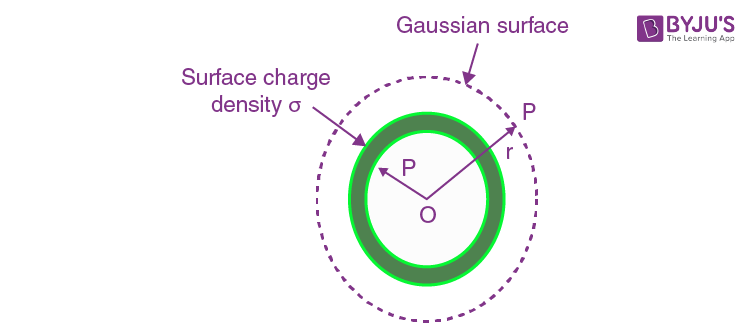Let us find out the electric field intensity at a point P outside or inside the shell.

Field Outside the Shell

We have to find the electric field intensity at a point P outside the spherical shell such that, OP = r.

Here, we take the Gaussian surface as a sphere of radius r.

Then, the electric field intensity is the same at every point of the Gaussian surface directed radially outwards,

So, according to Gauss’s theorem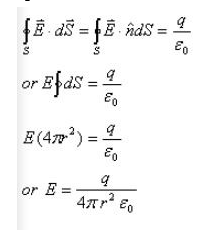So, it is clear that electric intensity at any point outside the spherical shell is such as if the entire charge is concentrated at the centre of the shell.

Field at the Surface of the Shell

Here, we have, r = R

Therefore,

E = q / (4 Π R2 εO)

If σ C/m2 is the charge density on the shell,

Then,

q=4 Π R2. σ

Therefore,

E = (4 Π R2. σ ) / (4 Π R2. εO) = σ /ε0

Field Inside the Shell

If point P lies inside the spherical shell, then the Gaussian surface is a surface of a sphere of radius r.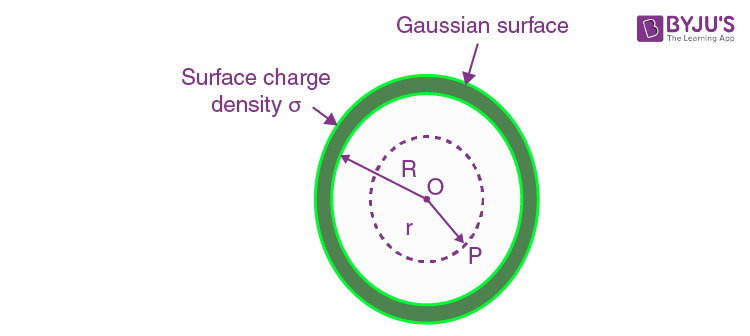As there is no charge inside the spherical shell, the Gaussian surface encloses no charge. That is q = 0.

Therefore,

E = 0

Hence, the field inside the spherical shell is always zero.

## Recommended Videos

### Electric Field – JEE Main Important Questions & Concepts### Electrostatics – Electric Field Lines and Electric Flux### Electrostatics – Electric Field and Electric Dipole### Electric Charges and Fields – Top 10 Most Important JEE Questions### Electric Charges and Fields One-shot Revision## Frequently Asked Questions on Electric Field

Q1

### What is an electric field?

The space surrounding an electric charge or a group of charges in which another charge experiences a force is called an electric field.

Q2

### When is the electric field said to be uniform?

If the force on the test charge is said to be the same at all points in a field, both in magnitude and direction, then the field is said to be uniform.

Q3

### When is the electric field said to be non-uniform?

If the force on the test charge varies from point to point in a field, then the field is said to be non-uniform.

Q4

### Does a charge experience a force due to its own field?

No, a charge will not experience any force due to its own field.

Test your Knowledge on electric field